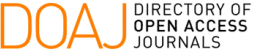### A Genetic Algorithm for Pointwise Source Reconstruction by the Method of Fundamental Solutions

J. R. Faria

#### Abstract

Inverse source reconstruction problems offer great potential for applications of interest to engineering, such as the identification of polluting sources, and to medicine, such as electroencephalography, to cite at least two relevant examples. From a mathematical point of view, the identification of a concentrated source (intensity and location) corresponds to the identification of the centroid (location) and size (intensity) of a distributed source. On the other hand, from a numerical point of view, it is observed that the use of domain discretization methods is intrinsically associated with the introduction of numerical noise in reconstruction algorithms, which is strongly inadvisable since inverse problems are reckoned to be ill-posed. The objective of this work is to explore, in the context of a Poisson problem and taking into account a numerical point of view, a new reconstruction algorithm based on the method of fundamental solutions, where a source point adequately represents the pointwise source within the domain. The inverse problem is reformulated as an optimization problem solved through a genetic algorithm. Finally, numerical examples are performed to analyze the accuracy of the proposed algorithm for two and three dimensions.

#### Keywords

Inverse Problems, Method of Fundamental Solutions, Genetic Algorithms, Source Reconstruction

#### Full Text:

PDF

DOI: https://doi.org/10.5540/tcam.2022.023.03.00401

### Refbacks

• There are currently no refbacks.

Trends in Computational and Applied Mathematics

A publication of the Brazilian Society of Applied and Computational Mathematics (SBMAC)

Indexed in: